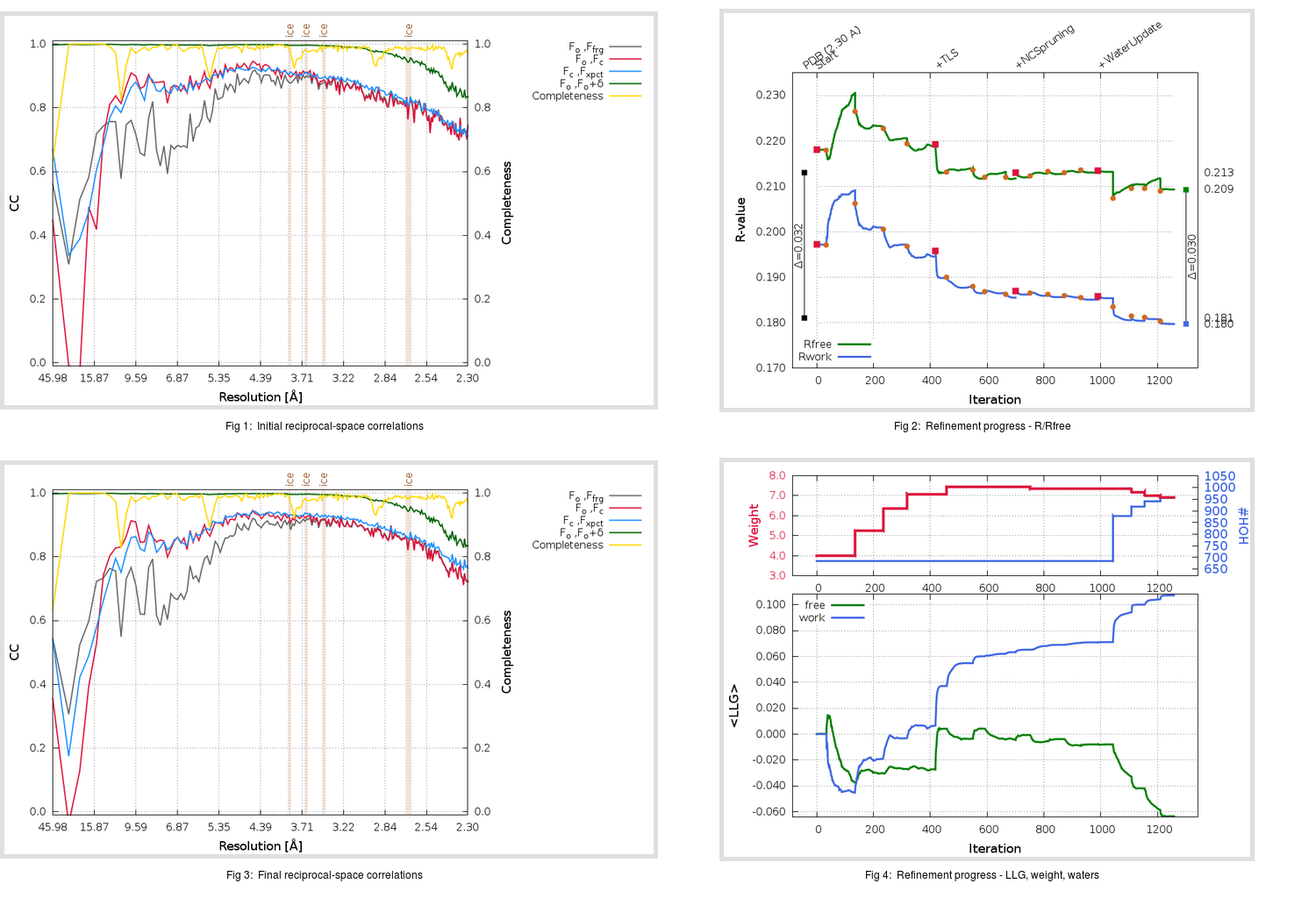Content:

```    Diffraction limits & principal axes of ellipsoid fitted to diffraction cut-off surface:
2.325         0.9893   0.0000  -0.1460       0.944 a* - 0.330 c*
2.418         0.0000   1.0000   0.0000       b*
2.233         0.1460   0.0000   0.9893       0.068 a* + 0.998 c*
```

## Deposited

` `
 Date deposited Date data collection Resolution R, Rfree 20190726 20180222 2.30 0.1800 0.2130

Molprobity (CCP4 7.0 version) summary:

```Ramachandran outliers =   0.00 %
favored =  97.50 %
Rotamer outliers      =   0.30 %
C-beta deviations     =     2
Clashscore            =   4.73
RMS(bonds)            =   0.0087
RMS(angles)           =   1.15
MolProbity score      =   1.34
Resolution            =   2.30
R-work                =   0.1800
R-free                =   0.2130
```

```Number of waters      =   685

<B> (all atoms) =   44.06 ( sd =   16.01 ) for      13170 non-hydrogen atoms
<B>   (protein) =   42.77 ( sd =   15.09 ) for      11878 non-hydrogen atoms
<B>     (water) =   44.79 ( sd =   10.45 ) for        685 non-hydrogen atoms
<B>    (others) =   68.52 ( sd =   18.59 ) for        607 non-hydrogen atoms

B min/max       (all non-hydrogen atoms) =   15.43 /  129.69
B min/max   (protein non-hydrogen atoms) =   16.83 /  129.69
B min/max     (water non-hydrogen atoms) =   15.43 /   74.14
B min/max     (other non-hydrogen atoms) =   26.87 /  116.40
```

## BUSTER (re-)refinement

` `

Molprobity (CCP4 7.0 version) summary:

```Ramachandran outliers =   0.13 %
favored =  97.77 %
Rotamer outliers      =   3.62 %
C-beta deviations     =     4
Clashscore            =   2.72
RMS(bonds)            =   0.0113
RMS(angles)           =   1.59
MolProbity score      =   1.54
Resolution            =   2.30
R-work                =   0.1798
R-free                =   0.2094
```

```Number of waters      =   956

<B> (all atoms) =   43.72 ( sd =   14.13 ) for      13441 non-hydrogen atoms
<B>   (protein) =   42.05 ( sd =   13.05 ) for      11878 non-hydrogen atoms
<B>     (water) =   49.06 ( sd =   11.73 ) for        956 non-hydrogen atoms
<B>    (others) =   67.90 ( sd =   13.71 ) for        607 non-hydrogen atoms

B min/max       (all non-hydrogen atoms) =   13.37 /  109.59
B min/max   (protein non-hydrogen atoms) =   19.45 /  109.59
B min/max     (water non-hydrogen atoms) =   13.37 /   99.69
B min/max     (other non-hydrogen atoms) =   32.53 /  101.38
```

Refinement progression:Results:

` `
 File Remark 6PXH_aB_refine.01_04_refine.pdb.gz exact refinement commands are in header 6PXH_aB_refine.01_04_refine.mtz.gz including original deposited data and several re-refinement map coefficients 6PXH_aB_refine.01_04_BUSTER_model.cif.gz including any non-standard compound restraints 6PXH_aB_refine.01_04_BUSTER_refln.cif.gz